# Out of Step (Z) Variables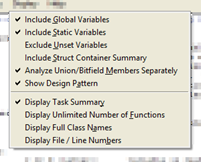The normal order of variable use in a task is for it to be set and then read. Out of Step (Z) Variables identifies global and static variables whose calculation order is read-then-set. In task-based software, this can cause stale values for a variable to be used in calculations.

Consider the following code. The variable globalA is initialized, and thus, even the first time taskA is invoked, it finds a value for globalA. However, taskA updates the value of globalA before completing. The next time taskA is invoked, the value it reads for globalA is that which existed at the end of the last invocation. This might be the desired behavior, but it could also be an indication of a programming error, where globalA should first be assigned (e.g. by reading a sensor).

 ```int globalA = 1; int subX() { int localX; localX = globalA; return localX; } void subY(int paramY) { globalA = paramY; } void taskA() { int localA, localB; localA = subX(); localB = localA; subY(localB); } ```

The Out of Step Variables report finds these read-then-set uses of global or static variables within tasks.

 ```Out-of-Step (Z) Variables (Read Before Being Set) Key: r or R (letter): variable is read s or S (letter): variable is set S or R (upper case): variable is used in current function s or r (lower case): variable is only used in called functions rs (order): variable is read in at least one path before being set sr (order): variable is set in all paths before being read r* (order): variable is read first in at least one path; it is set and read in various orders s* (order): variable is set first in all paths where it is read; it is set and read in various orders thereafter RO (PTN): pattern of variable use across all tasks is read only Z1 (PTN): pattern is status variable (only set to constants) Z2 (PTN): pattern is aggregation variable (assignments involve its own value) Z3 (PTN): pattern is external data variable (array set to constants and likely written to externally like DMA) Settings: Global Variables: displayed Static Variables: displayed Unset Variables: displayed Struct Container Summary: omitted Union/Bitfield Members: separate Show Z-var Design Pattern: displayed Task Definitions Tasks are from User Defined Tasks Name Members Graph Root Task A 3 [+] taskA Variable PTN Func1 Func2 Func3 (order of calls) Task: Task A taskA . subX . . subY -------------------------------------------------- = = = globalA .......................................... rs R S ```

### Design Patterns

Some of the identified out-of-step variable usage in your software might conform to standard design patterns that can be considered acceptable usage. The PTN column of the report indicates when the use of a variable fits one of these patterns. You can quickly confirm such usage and then focus your review on the reported variable uses that don't match the patterns.

#### Z1 - Status Variable

The Z1 pattern refers to a global variable that is used to tell the task what status the system was in previously. The task reads the variable first in an if statement and then sets the status to another value. The status variable is never used in the calculation of any other variable.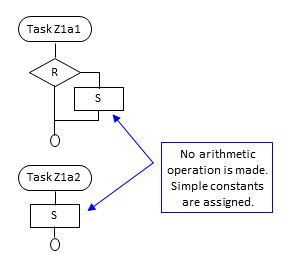```int Status = 0;

if (Status) {
/* Status is read first in "if" or
"switch" but not in a calculation */
SomeAction();
Status = 0; /* Status set to constant */
}
}

int thisHappened;
thisHappened = SomeValue();
if (thisHappened) {
Status = 1;
}
}
```

A second case of Z1 pattern involves task requests. A task or external process sets a variable in order to cause an action in a second task. The second task checks the variable to see if there is something to do. If so, it performs the action and resets the action request variable.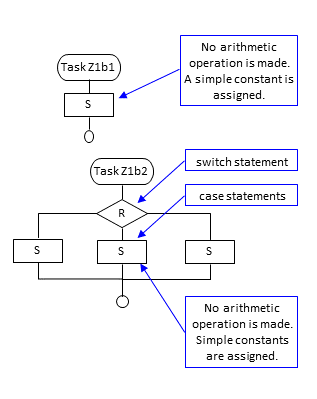```int Action = 0;
int ActionParam;

Action = 1;/* task requests action */
ActionParam = SomeValue();
}

switch (Action) {
/* task checks for action */
case 1:
SomeAction1(ActionParam);
Action = 0; /* resets action code */
break;
case 2:
SomeAction2(ActionParam);
Action = 0;
break;
}
}
```

 Type of Variable Single (no array, no struct member); int or enum Tasks Using Variable One or multiple Use Patterns Either RS or S Conditions on Reads In if or switch condition only Conditions on Sets Only constants are assigned

#### Z2 - Aggregation Variable

The Z2 pattern identifies the situation where one task aggregates values in a variable over several cycles. For example, the task might add the values to the same variable in every cycle, or insert the values into an array and thereby increase the index in every cycle, or check if the value tracked in the variable is greater than a maximum. In each of these cases, the task will first read the variable that is used to aggregate the values and then set it. A second task will eventually read this aggregated variable and use it in its calculations. The second task might then reset it so that a new aggregation cycle can start.

Example Z2a with an out-of-step single variable (scalar) where value is added to aggregate in every cycle: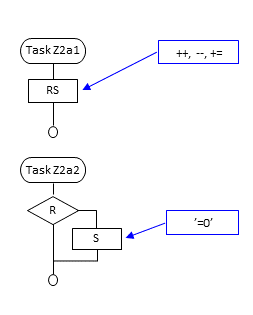```int AggregateCounter = 0;
double Aggregate = 0.0;

{
Aggregate += SomeFloatValue();
AggregateCounter++;
}

if (AggregateCounter < 10) {
from time to time */
double Calc;
Calc = SomeCalc(Aggregate);

/* resets aggregate variables */
AggregateCounter = 0;
Aggregate = 0.0;
}
}
```

Example Z2b with an out-of-step struct members where maximum value is tracked: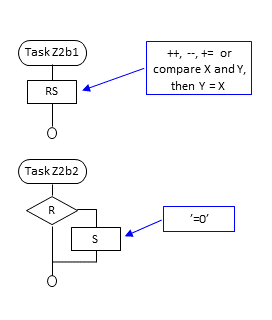```#define AGGRB 100
struct Aggr {
int AC;
double Max;
} GlobAggr = {0, 0.0};

double val = SomeFloatValue();
if (val > GlobAggr.Max) {
GlobAggr.Max = val;
}
GlobAggr.AC++;
}

if (GlobAggr.AC >= AGGRB) {
Display(GlobAggr.Max);
/* resets aggregate variables */
GlobAggr.AC = 0;
GlobAggr.Max = 0.0;
}
}
```

Example Z2c with an out-of-step array where values are inserted in every cycle within a single task: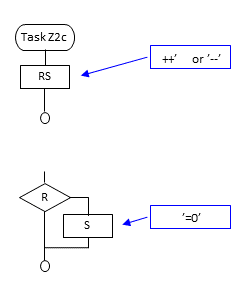```#define AGGRC 100
int AC = 0;
double AggregateC[AGGRC]; /* Z-var */
double SomeCalc(double[]);

if (AC >= AGGRC) {
/* task evaluates aggregated value */
double Calc;
Calc = SomeCalc(AggregateC);

/* resets aggregate variables */
AC = 0;
}else {
if (AC > 0) {
/* track two point moving average */
AggregateC[AC] = (AggregateC[AC-1] +
SomeFloatValue()) / 2;
}else {
AggregateC[AC] = SomeFloatValue();
}
AC++;
}
}
```

 Type of Variable Single, array, struct member; any type Tasks Using Variable One or multiple Use Patterns RS or S Conditions on Reads Either operation that involves its own value (++, --, +=, -=, &=, compare and set) or in if condition or calculation followed by reset to constant value Conditions on Sets Either operation that involves its own value (++, --, +=, -=, &=) or reset to constant value Further Notes Aggregation count-up and reset can either happen in same task or in separate tasks

#### Z3 - External Data Variable

The Z3 pattern identifies situations such as where sensor hardware writes directly to memory and the task reads the memory. Normally, this would not be flagged as an out-of-step variable in the report because the access would be read-only. However, in some designs, the task also resets (part of) the memory when it is done. In such cases, the variable usage is listed as out-of-step and indicated with the Z3 flag.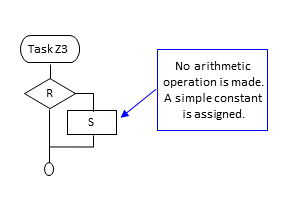```/* variable for DMA area */
volatile int DMAVar;